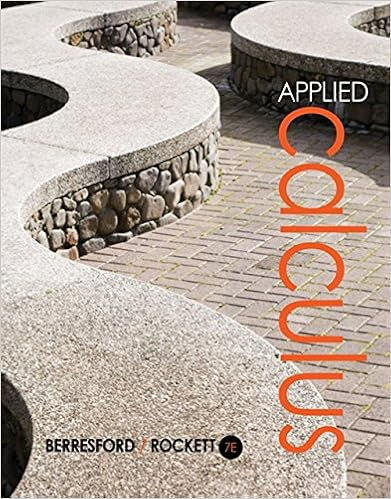# Time you move one column to the right the place value

• 612
• 67% (9) 6 out of 9 people found this document helpful

This preview shows page 352 - 356 out of 612 pages.

##### We have textbook solutions for you!
The document you are viewing contains questions related to this textbook.The document you are viewing contains questions related to this textbook.
Chapter 1 / Exercise 18
Applied Calculus
Berresford/RockettExpert Verified
time you move one column to the right, the place value is 1/10 of the placevalue of the preceding column.Now, consider thedecimal number12.3456, which consists of three parts:the whole number part, the decimal point, and the fractional part.
##### We have textbook solutions for you!
The document you are viewing contains questions related to this textbook.The document you are viewing contains questions related to this textbook.
Chapter 1 / Exercise 18
Applied Calculus
Berresford/RockettExpert Verified
5.1.INTRODUCTION TO DECIMALS34312.3456Whole number partDecimal pointFractional partThe whole number part of the decimal number is the part that lies strictlyto the left of the decimal point, and the place value of each digit in the wholenumber part is given by the columns shown in Table5.1.The fractional part of the decimal number is the part that lies strictly tothe right of the decimal point. As we saw in Table5.1, each column has a valueequal to 1/10 of the value of the column that lies to its immediate left. Thus,it should come as no surprise that:The first column to the right of the decimal point has place value 1/10(tenths).The second column to the right of the decimal point has place value 1/100(hundredths).The third column to the right of the decimal point has place value 1/1000(thousandths).The fourth column to the right of the decimal point has place value1/10000 (ten-thousandths).These results are summarized for the decimal number 12.3456 in Table5.2.12.3456tensonesdecimal pointtenthshundredthsthousandthsten-thousandths101.1/101/1001/10001/10000Table 5.2: Place value.
344CHAPTER 5.DECIMALSPronouncing Decimal NumbersThe decimal number 12.3456 is made up of 1 ten, 2 ones, 3 tenths, 4 hundredths,5 thousandths, and 6 ten-thousandths (see Table5.2), and can be written inexpanded formas12.3456 = 10 + 2 +310+4100+51000+610000.Note that the whole numbers can be combined and the fractions can be writtenwith a common denominator and summed.12.3456 = 12 +3·100010·1000+4·100100·100+5·101000·10+610000= 12 +300010000+40010000+5010000+610000= 12 +345610000The result tells us how to pronounce the number 12.3456.It is pronounced“twelve and three thousand, four hundred fifty-six ten-thousandths.”You Try It!EXAMPLE 1.Place the decimal number 1,234.56 in expanded form, thenPlace the decimal number3,502.23 in expanded form,then combine the wholenumber part and sum thefractional part over acommon denominatorcombine the whole number part and sum the fractional part over a commondenominator. Use the result to help pronounce the decimal number.Solution.In expanded form,1,234.56 = 1,000 + 200 + 30 + 4 +510+6100Sum the whole number parts. Express the fractional parts as equivalent frac-tions and combine over one common denominator.= 1,234 +5·1010·10+6100= 1,234 +50100+6100= 1,234 +56100Hence, 1,234.56 is pronounced “one thousand, two hundred thirty-four andfifty-six hundredths.”Answer:3,502 +23100
5.1.INTRODUCTION TO DECIMALS345You Try It!EXAMPLE 2.Place the decimal number 56.128 in expanded form, thenPlace the decimal number235.568 in expanded form,then combine the wholenumber part and sum thefractional part over acommon denominatorcombine the whole number part and sum the fractional part over a commondenominator. Use the result to help pronounce the decimal number.
•••